Published

# Temperature Measurement

Two Particle Argon That was created to measure the inside temperature and outside temperature.

IntermediateFull instructions provided7 hours5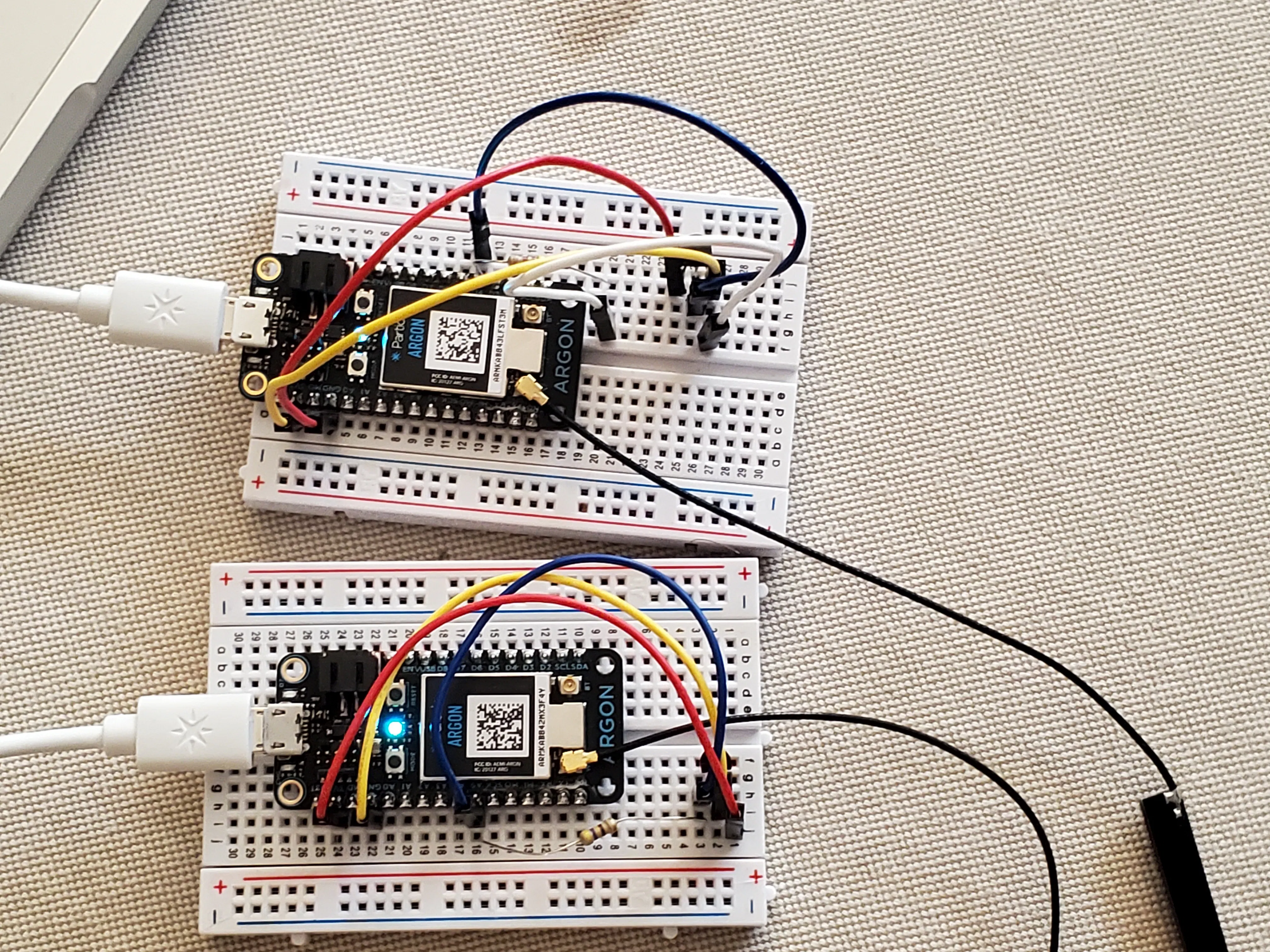## Things used in this project

### Hardware components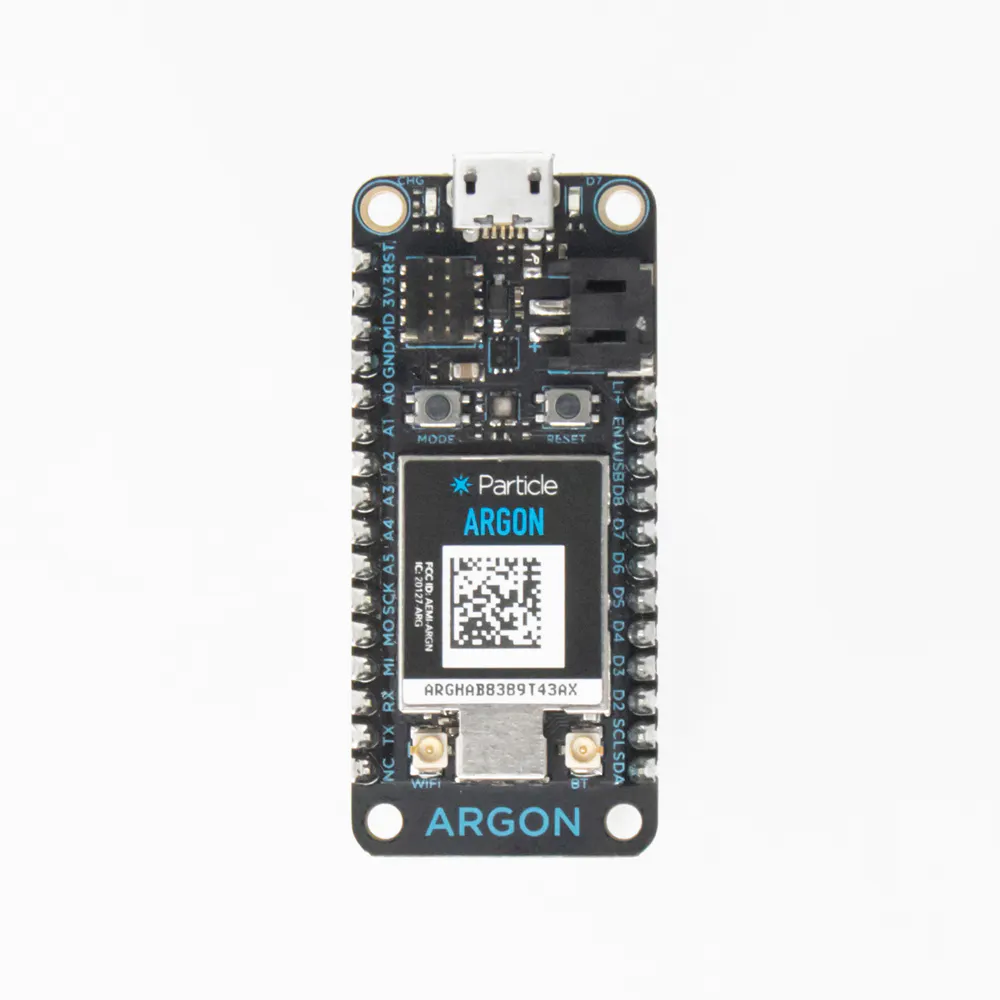Particle Argon
×2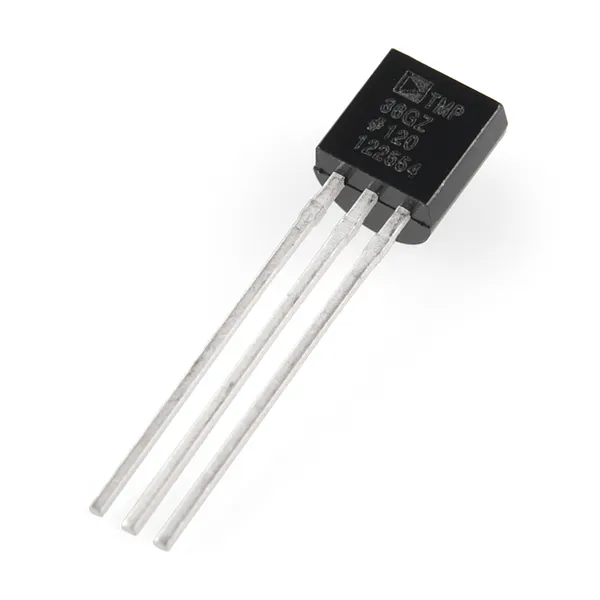Temperature Sensor
×2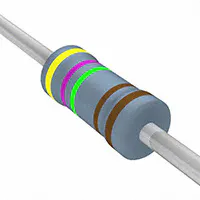Resistor 4.75k ohm
×2×2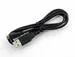USB-A to Micro-USB Cable
×2Jumper wires (generic)
×10

### Software apps and online servicesParticle Build Web IDE

## Schematics

### Inside Temperature Circuit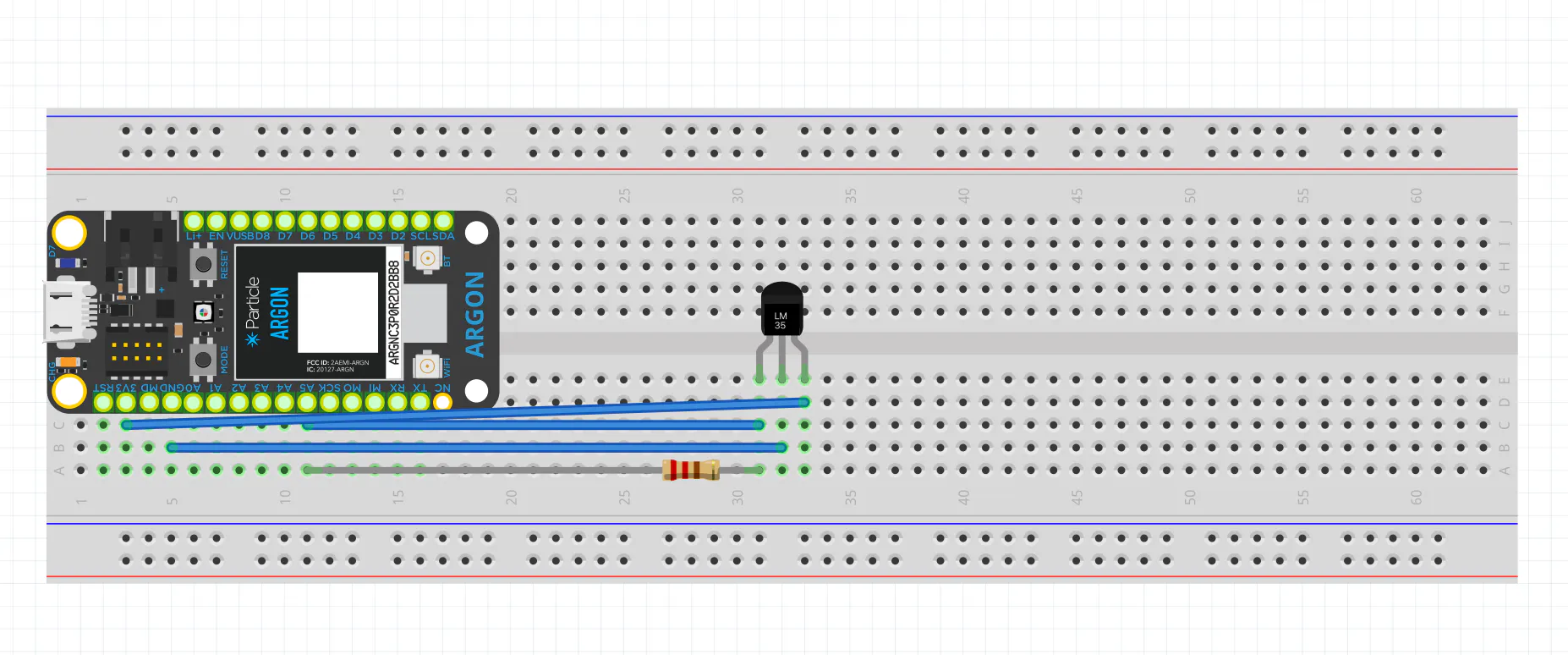### Outside Temperature Circuit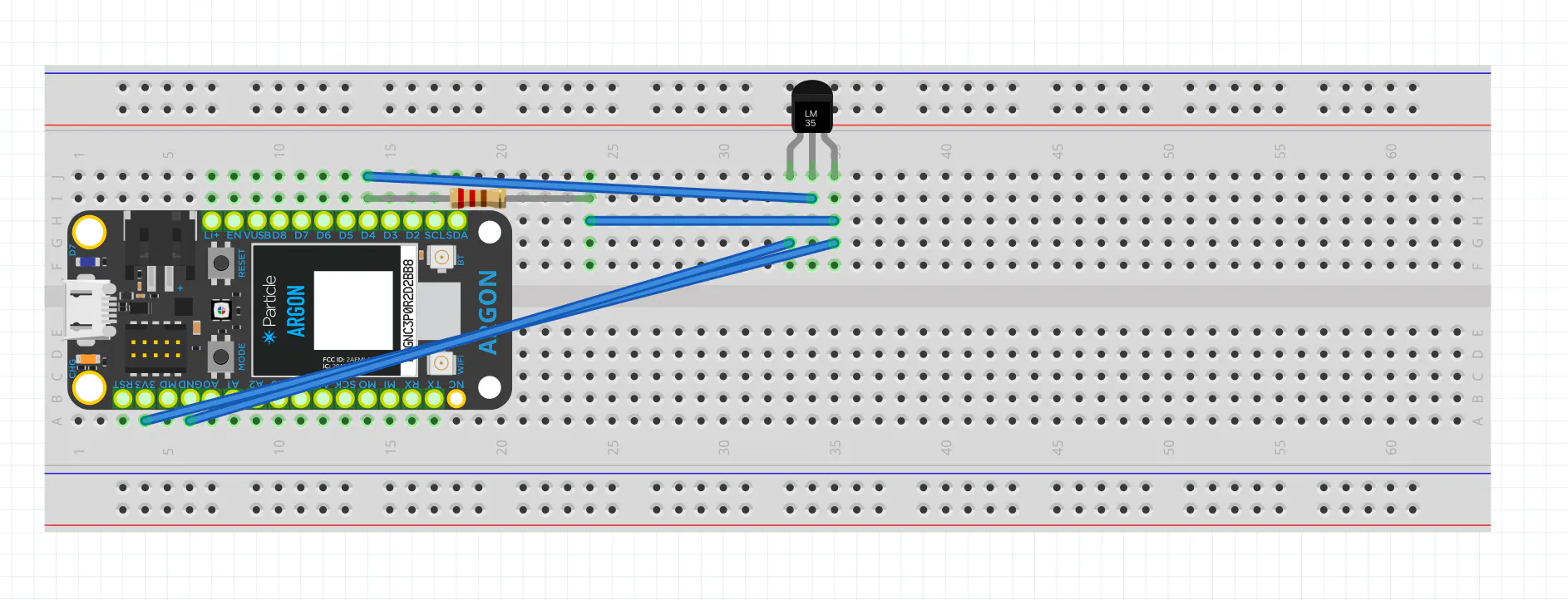## Code

### Inside Temperature

C/C++
```int temperaturePin = A5; //temperature sensor pin
int tempHigh = 28; //top value in range of temperature values
int tempLow = 24; //bottom value in range of temperature values

void setup() {

//set temperature and push-button
pinMode(temperaturePin, INPUT);

//debugging
Serial.begin(9600);
}

void loop() {
double temperature = (analogRead(temperaturePin) * 3.3) / 4095;  //getting the voltage reading from the temperature sensor
temperature = (temperature - 0.5) * 100; //turn the voltage into a Celcius reading
Serial.println(temperature); //print temperature to serial for debugging
// Publish data
Particle.publish("temperature2807112", String(temperature), PRIVATE);
digitalWrite(D7,HIGH);
delay(2000);
digitalWrite(D7,LOW);

}
```

### Outside Temperature

C/C++
```#include <OneWire.h>

#include <OneWire.h>

OneWire ds = OneWire(D4);  // 1-wire signal on pin D4

unsigned long lastUpdate = 0;

float lastTemp;

int led = D7;  // The on-board LED
int temperaturePin = D5; //temperature sensor pin
void setup() {
Serial.begin(9600);
// Set up 'power' pins, comment out if not used!
pinMode(D3, OUTPUT);
pinMode(D5, OUTPUT);
digitalWrite(D3, LOW);
digitalWrite(D5, HIGH);
}
void loop() {
byte i;
byte present = 0;
byte type_s;
byte data;
float celsius, fahrenheit;

Serial.println();
ds.reset_search();
delay(250);
return;
}
// first the returned address is printed

Serial.print("ROM =");
for( i = 0; i < 8; i++) {
Serial.write(' ');
}

// second the CRC is checked, on fail,

Serial.println("CRC is not valid!");
return;
}
Serial.println();

// the first ROM byte indicates which chip
case 0x10:
Serial.println("  Chip = DS1820/DS18S20");
type_s = 1;
break;
case 0x28:
Serial.println("  Chip = DS18B20");
type_s = 0;
break;
case 0x22:
Serial.println("  Chip = DS1822");
type_s = 0;
break;
case 0x26:
Serial.println("  Chip = DS2438");
type_s = 2;
break;
default:
Serial.println("Unknown device type.");
return;
}

ds.reset();               // reset the 1-wire bus
ds.write(0x44, 0);        // begins conversion
delay(1000);              //wait 1 sec
present = ds.reset();
ds.write(0xB8,0);         // Recall Memory 0
ds.write(0x00,0);         // Recall Memory 0

present = ds.reset();
if (type_s == 2) {
ds.write(0x00,0);       // The DS2438 needs a page# to read
}

// transfer and print the values

Serial.print("  Data = ");
Serial.print(present, HEX);
Serial.print(" ");
for ( i = 0; i < 9; i++) {           // we need 9 bytes
Serial.print(data[i], HEX);
Serial.print(" ");
}
Serial.print(" CRC=");
Serial.print(OneWire::crc8(data, 8), HEX);
Serial.println();

// Convert the data to actual temperature
// because the result is a 16 bit signed integer, it should
// be stored to an "int16_t" type, which is always 16 bits
// even when compiled on a 32 bit processor.
int16_t raw = (data << 8) | data;
if (type_s == 2) raw = (data << 8) | data;
byte cfg = (data & 0x60);

switch (type_s) {
case 1:
raw = raw << 3; // 9 bit resolution default
if (data == 0x10) {
// "count remain" gives full 12 bit resolution
raw = (raw & 0xFFF0) + 12 - data;
}
celsius = (float)raw * 0.0625;
break;
case 0:
// at lower res, the low bits are undefined, so let's zero them
if (cfg == 0x00) raw = raw & ~7;  // 9 bit resolution, 93.75 ms
if (cfg == 0x20) raw = raw & ~3; // 10 bit res, 187.5 ms
if (cfg == 0x40) raw = raw & ~1; // 11 bit res, 375 ms
// default is 12 bit resolution, 750 ms conversion time
celsius = (float)raw * 0.0625;
break;

case 2:
data = (data >> 3) & 0x1f;
if (data > 127) {
celsius = (float)data - ((float)data * .03125);
}else{
celsius = (float)data + ((float)data * .03125);
}
}

// remove random errors
if((((celsius <= 0 && celsius > -1) && lastTemp > 5)) || celsius > 125) {
celsius = lastTemp;
}

fahrenheit = celsius * 1.8 + 32.0;
lastTemp = celsius;
Serial.print("  Temperature = ");
Serial.print(celsius);
Serial.print(" Celsius, ");
Serial.print(fahrenheit);
Serial.println(" Fahrenheit");

// now that we have the readings, we can publish them to the cloud
digitalWrite(D7, HIGH);

Particle.publish("temperature", String(fahrenheit), PRIVATE); // publish to cloud
delay(5000);// 5 second delay
digitalWrite(D7, LOW);
}
```

## Credits

### Ali Alkhabbaz

0 projects • 0 followers

### Omar Alqahtani

0 projects • 0 followers
Thanks to Megan Dietrich, Michael Coffey.